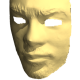Ask Your Question

# Pose Estimation and Feature Detection

Hi all,

I am trying to make a fiducial (QR marker) based pose estimation program which would be able to track multiple markers. I have started by building upon the OpenCV documentation example.

To do so I started by finding key points and descriptors using the SIFT algorithm. Then a used Brute Force matching to find good matches (used ratio test as well).

Now I need to use solvePnpRansac to find the pose estimation. However, as per my understanding the pose estimation algorithm requires coordinates between the real world object and 2D scene. How can I get the coordinates of the keypoints which have been matched by the SIFT algorithm?

Thanks.

edit retag close merge delete

## 1 answer

Sort by » oldest newest most votedDepends on your application. Do you have a set of fixed markers or a several markers which can move around the scene ?

As you said, solvePNP will give the RT matrix of a camera given the 3D coordinates of some points on the image, and these coordinates have to be known by another method.

For augmented reality with markers, the concept is that you have an idea about the real-world size of the markers a priori, so, for instance, for a 10cm square marker you can say that the coordinates of its corners are (0,0,0), (0.1,0,0), (0,0.1,0), (0.1,0.1,0). Once you have detected it, solvePNP will give you the relative pose of the camera towards this marker.

Note that the RT matrix is the transform that converts absolute world coordinates to relative coordinates towards the camera. So, if the centre of the marker is the position P = (0.05,0.05,0,1.0) (homogeneous coordinates) will be the centre of the marker, and its relative position in relation to the camera will be RT*P. This can be also be used to determine the marker orientation.

Likewise, if you want draw something as overlay over the marker (augmented reality), you can use the coordinates of the marker as the "world coordinates", and render the overlay based in the computed camera pose.

That said, if you have several mobile markers, you have to compute for each marker the relative pose of the camera from it with separated calls of solvePNP.

Note that if the appearance of the markers is known, and you don't have their real-world size, you will have to assign a defined size in an arbitrary unit, since there is a infinite number of possible sizes + 3D positions which will have the same appearance in the camera.

Important: RT is a 4x4 Matrix and P is a 4x1 matrix (x,y,z,w) where w is 1.0 (homogeneous coordinates). Solve PNP will give you the the euler angles R', and a translation matrix T'. You should compute the rotation matrix R (3x3) using cv::Rodrigues. I use the following procedure to compute RT from rvec and tvec from solvePNP :

void RvecTvecToRT(cv::Mat& Rvec, cv::Mat& Tvec, cv::Mat& RT)
{

RT = cv::Mat::eye(4,4,CV_64F); //identity matrix
cv::Mat R;
cv::Rodrigues(Rvec, R);
//We store the R and T that transforms from World to Camera coordinates
for(int i = 0; i < 3; i++) {
RT.at<double>(i,3) = Tvec.at<double>(i,0);
for(int j = 0; j < 3; j++) {
RT.at<double>(i,j) = R.at<double>(i,j);
}
}

}


Based in your comment, it is very similar with what I had implemented such thing long time ago, using pictures as AR markers.

Basically, as pre-processing step, you have first to compute the keypoints and associated descriptors for each AR marker. That is, for a marker, you will have a set of ...

more

## Comments

Dear Saracchini,

Thank you for your reply. I want to find the global pose of an object which has multiple uniquely identifiable markers attached to it at different angles/view points.

As you stated, my idea is to pass the solvePnp algorithm the real world (3D) co-ordinates of the identifiable key points. As for the 2D points it would be the co-ordinates of the keypoints which have been positively matched by brute force. I want to know how can I extract the marker identity and 2D co-ordinates of the matched key points for further use in PnP algorithm.

In actual I want to get an extremely stable sort of ARToolKit type functionality.

Thanks for your patience and help.

Official site

GitHub

Wiki

Documentation

## Stats

Asked: 2014-11-09 11:53:52 -0500

Seen: 1,333 times

Last updated: Nov 12 '14